# Quick learning in minutes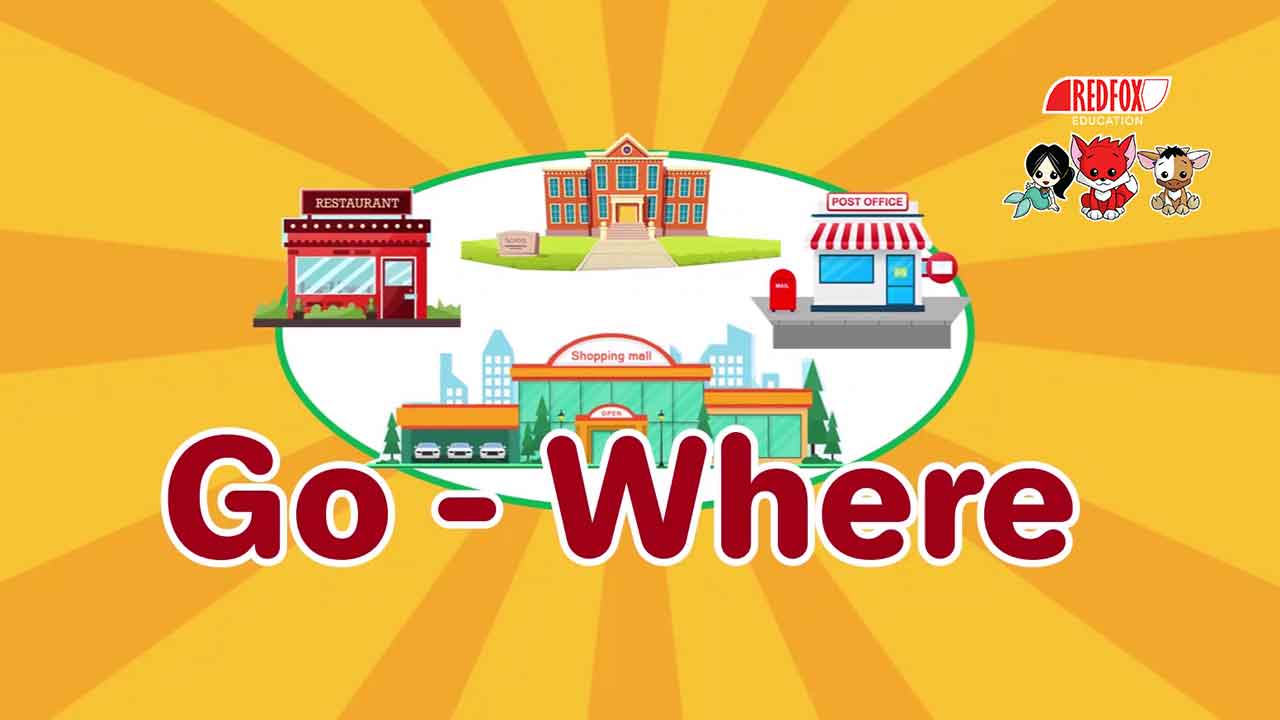##### Let's learn about the Go -Where
Let's learn about the Go -Where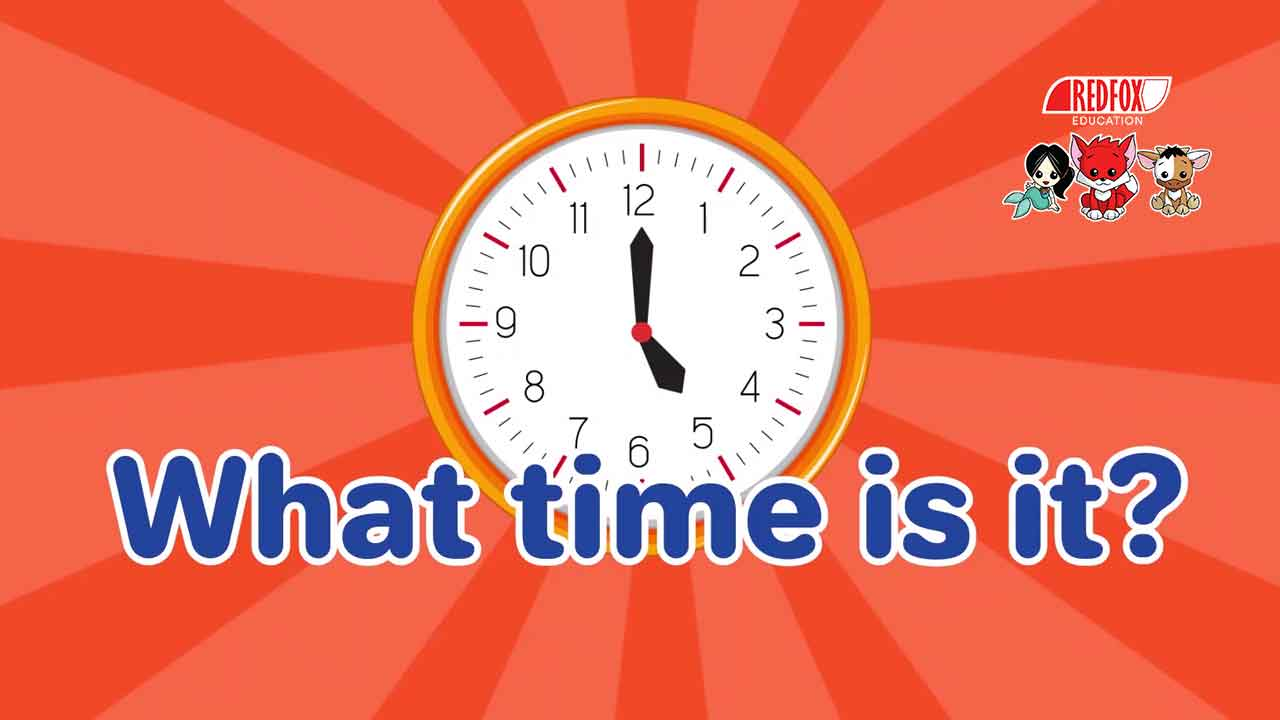##### Let's learn about the Time - What time is it
Let's learn about the Time - What time is it. What's time right now?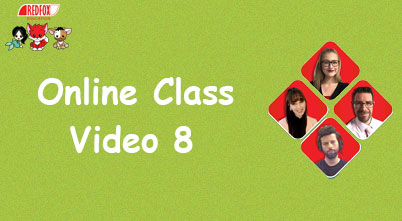##### Online Class 8
Online Class 8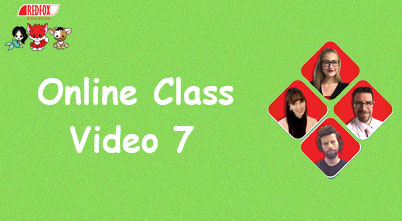##### Online Class 7
Online Class 7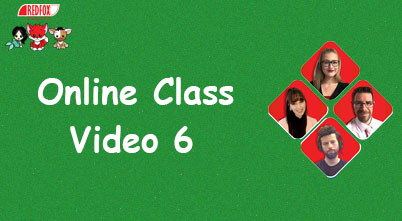##### Online Class 6
Online Class 6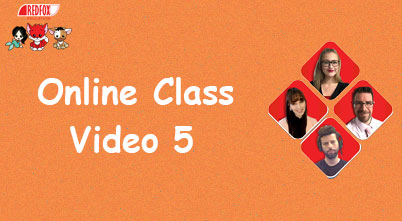##### Online Class 5
Online Class 5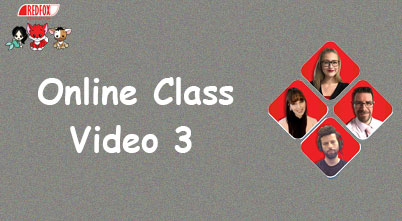##### Online Class 3
Online Class 3##### Online Class 1
Online Class 1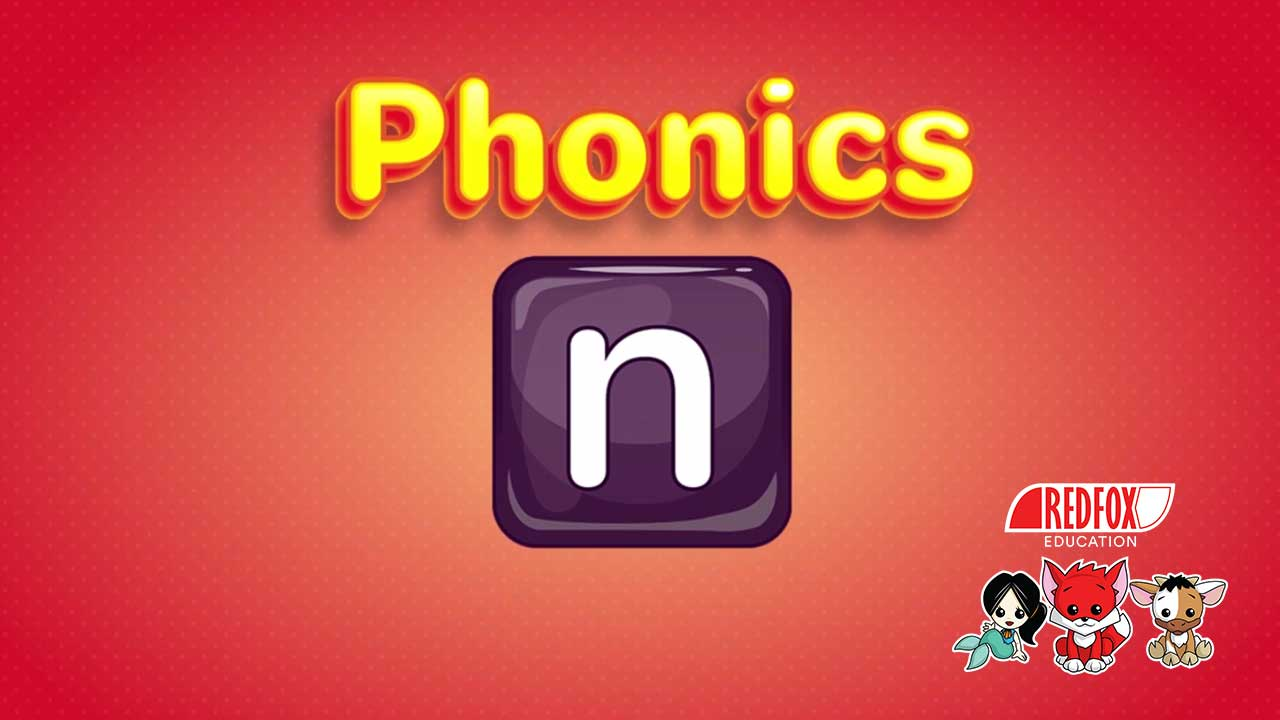##### Let's learn about the Phonics n
Let's learn about the Phonics n. What is Phonics n?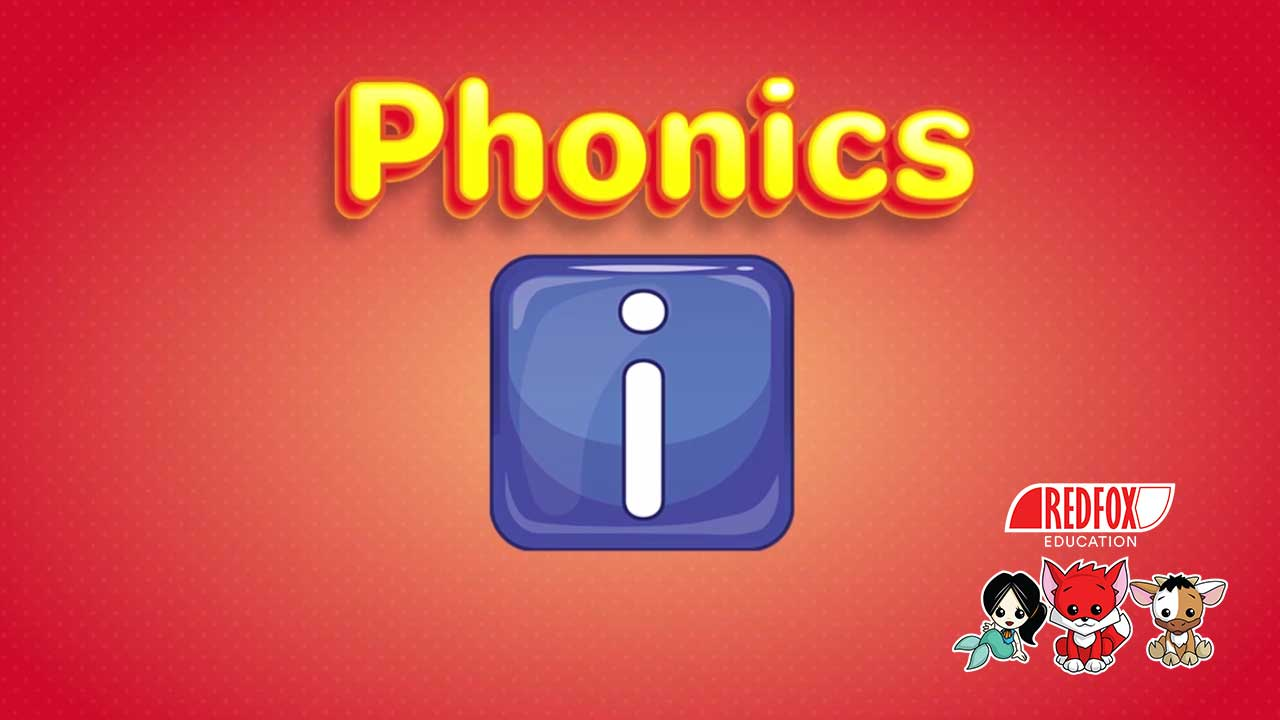##### Let's learn about the Phonics i
Let's learn about the Phonics i. What is Phonics i?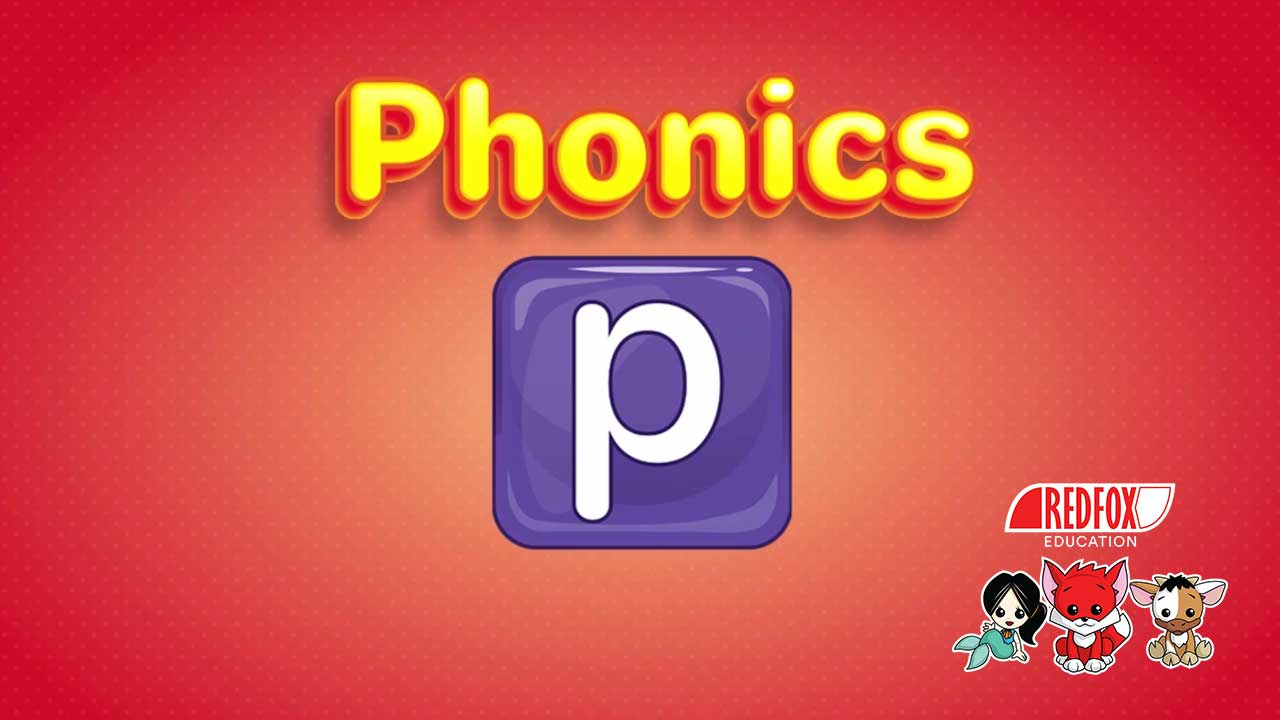##### Let's learn about the Phonics p
Let's learn about the Phonics p. What is Phonics p?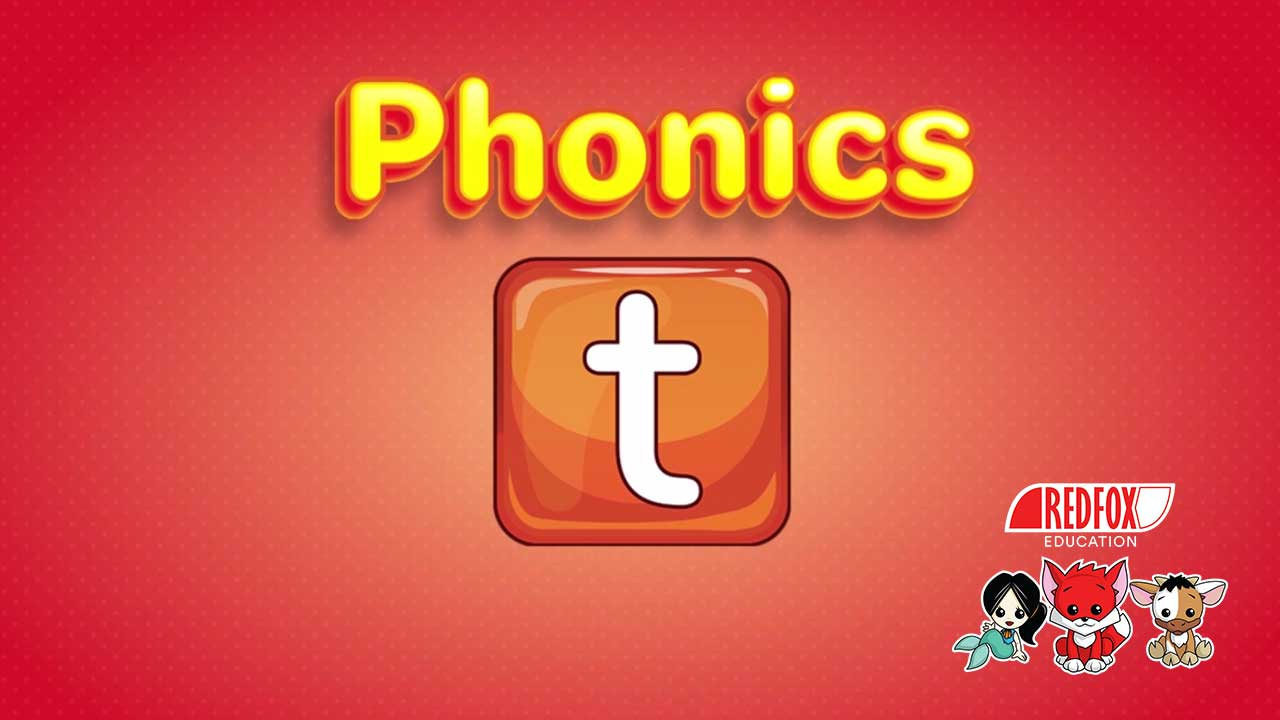##### Let's learn about the Phonics t
Let's learn about the Phonics t. What is Phonics t?##### Let's learn about the Phonics a
Let's learn about the Phonics a. What is Phonics a?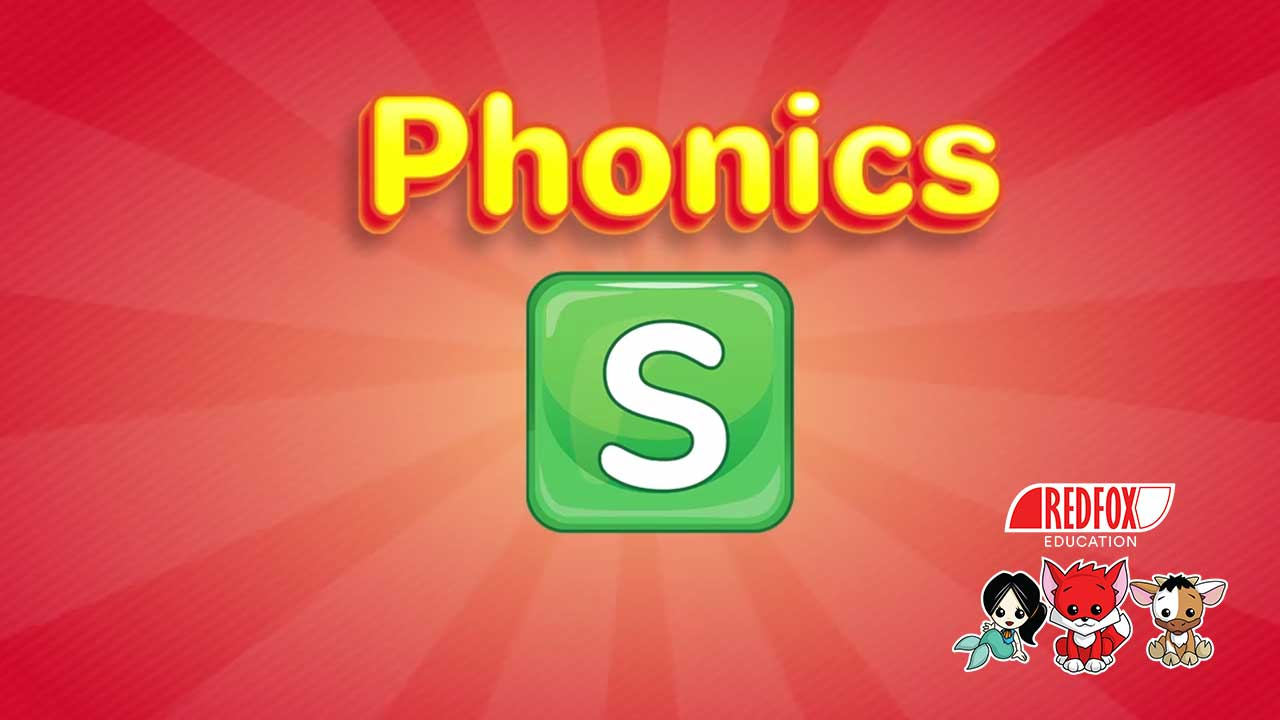##### Let's learn about the Phonics s
Let's learn about the Phonics s. What is Phonics s?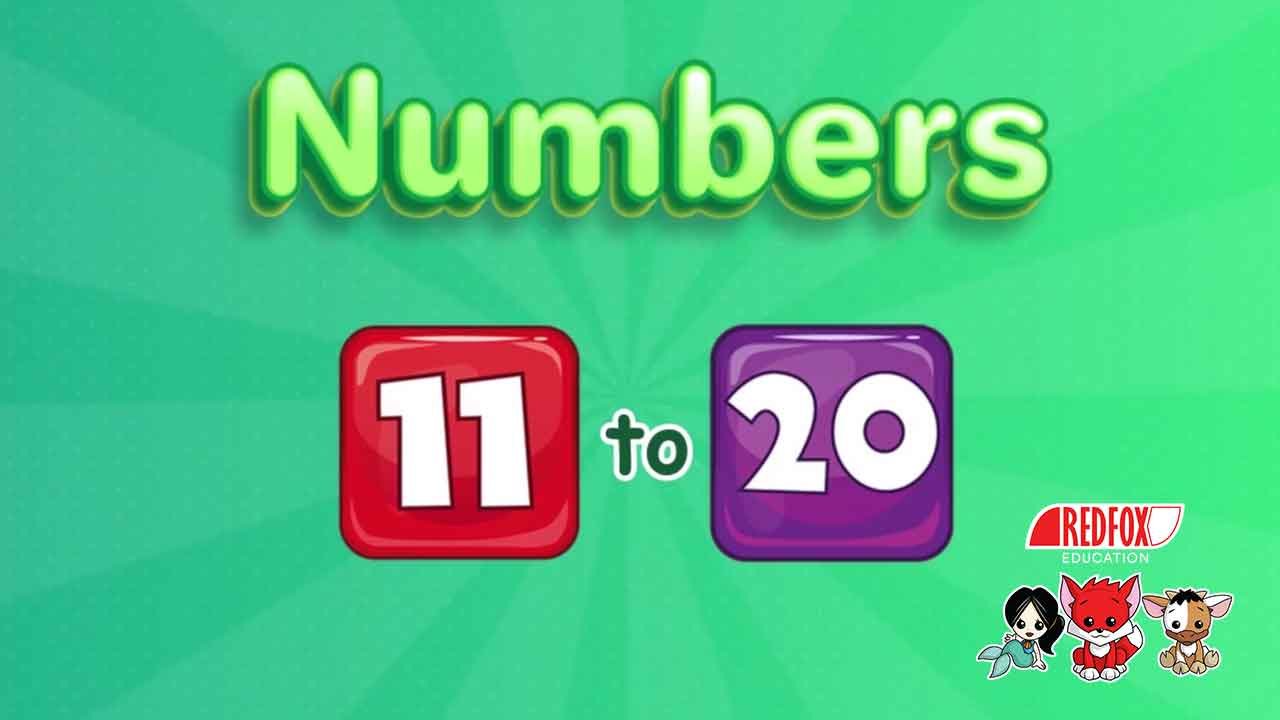##### Let's learn about the Numbers 11 to 20
Let's learn about the Numbers 11 to 20. What are they?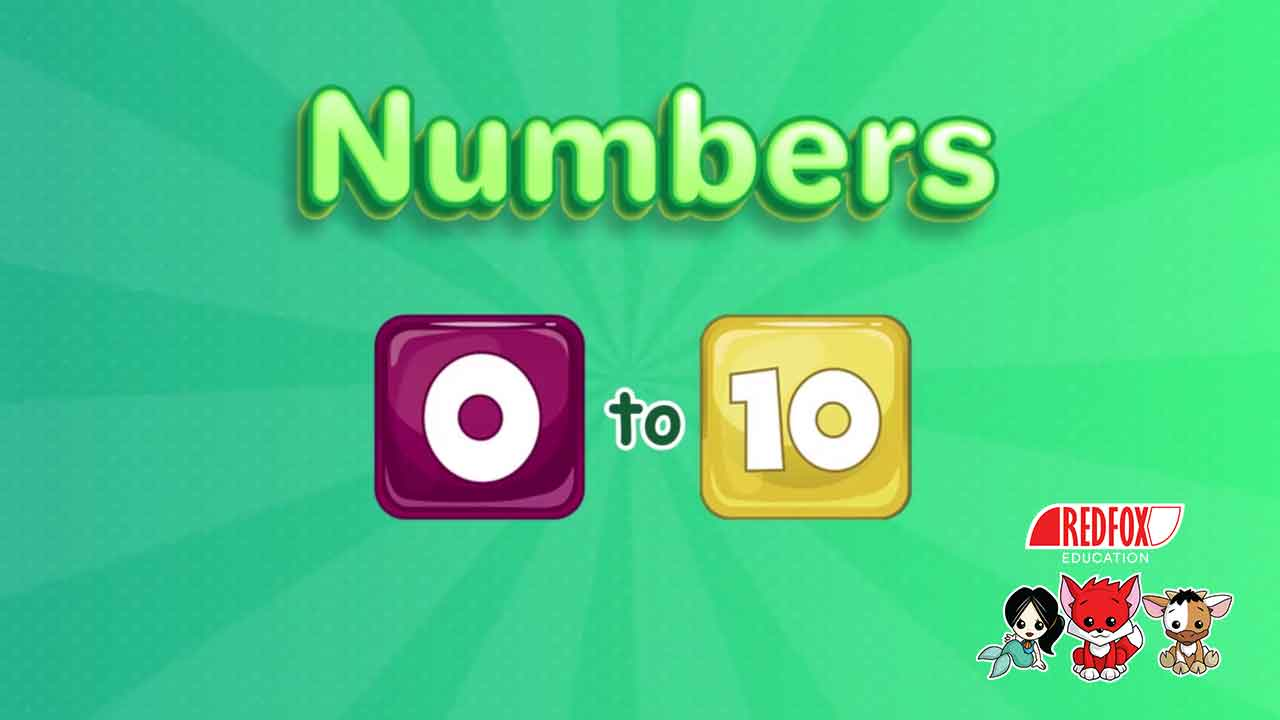##### Let's learn about the Numbers 0 to 10
Let's learn about the Numbers 0 to 10. What are they?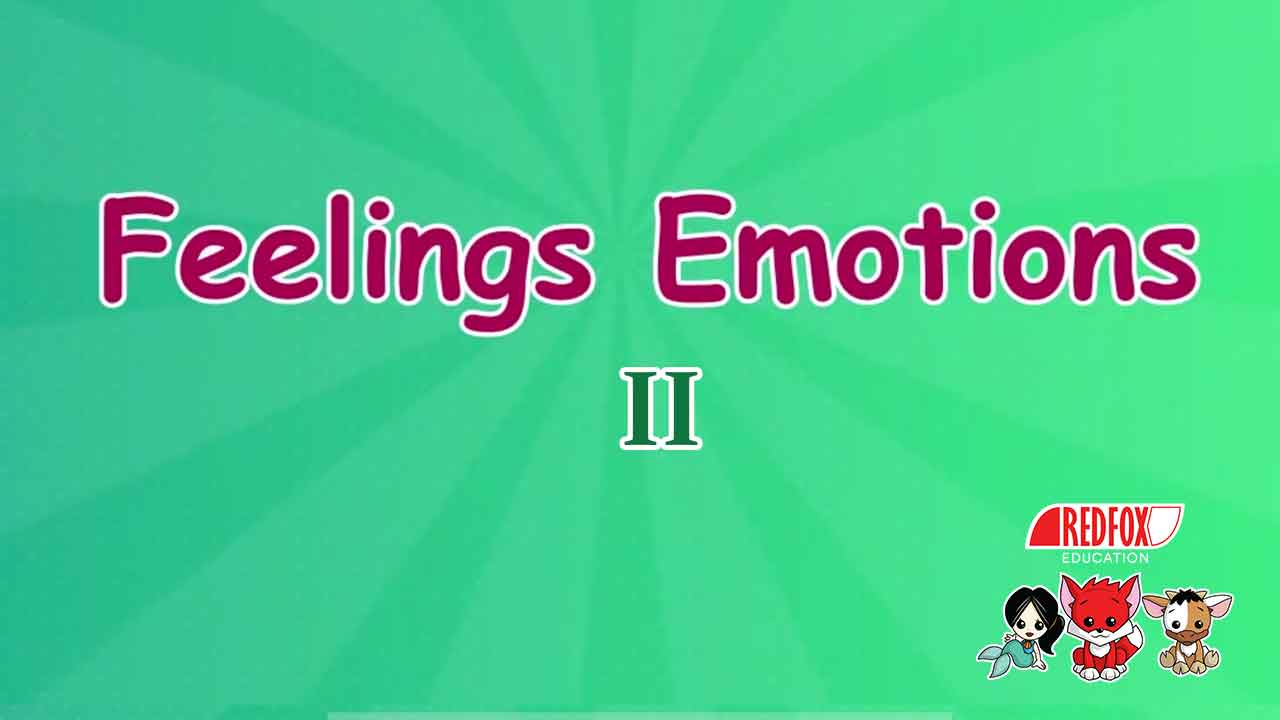##### Let's learn about the Feelings and Emotions II
Let's learn about the Feelings and Emotions II. What are they?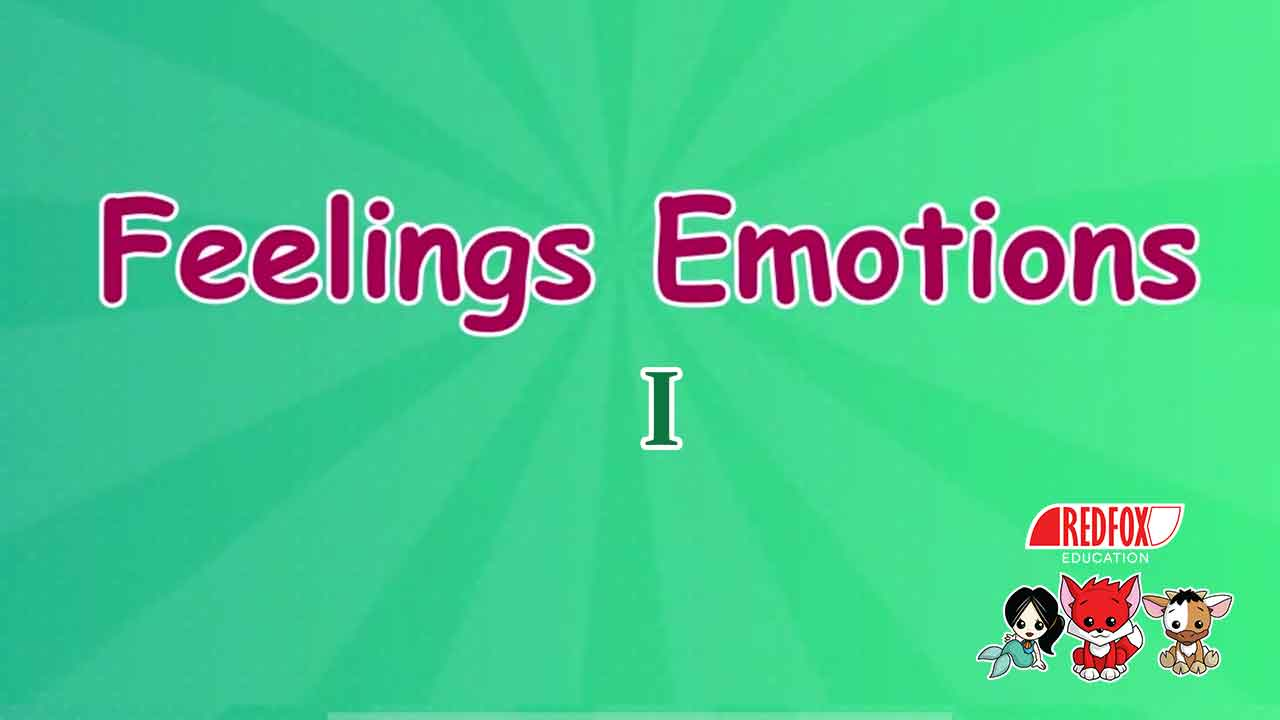##### Let's learn about the Feelings and Emotions I
Let's learn about the Feelings and Emotions I. What are they?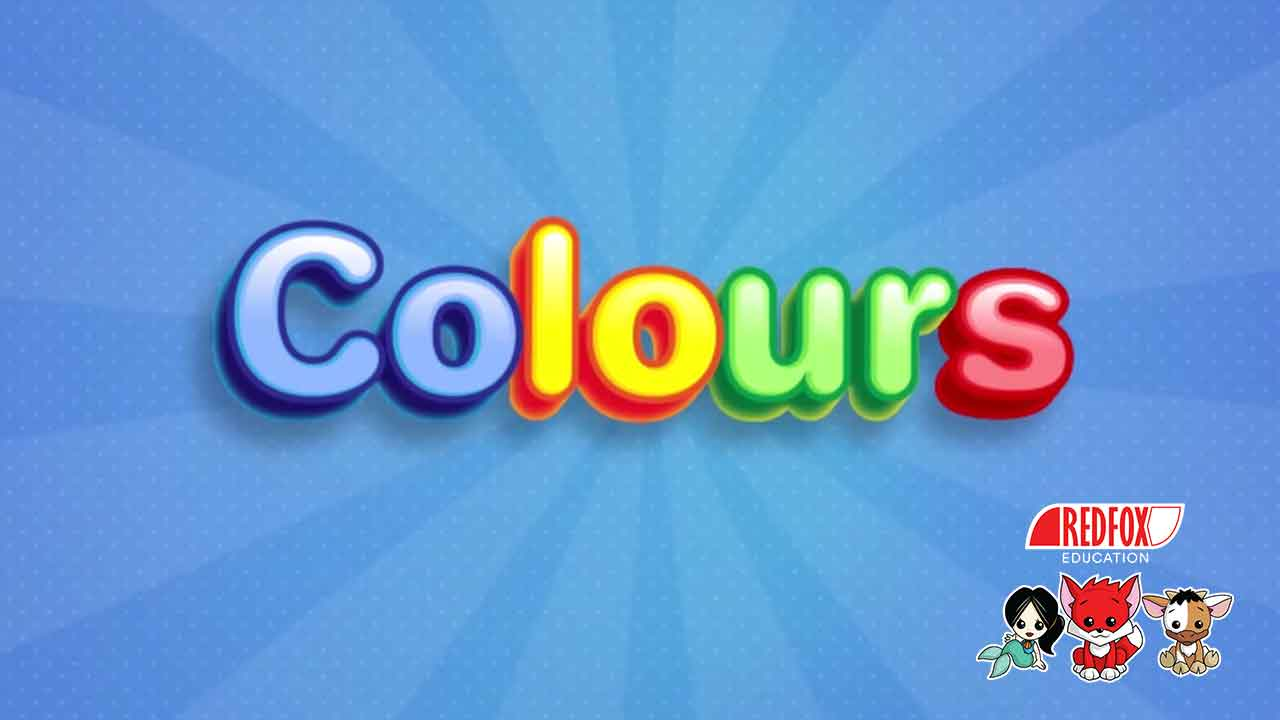##### Let's learn about the Colours
Let's learn about the Colours. What are the colours?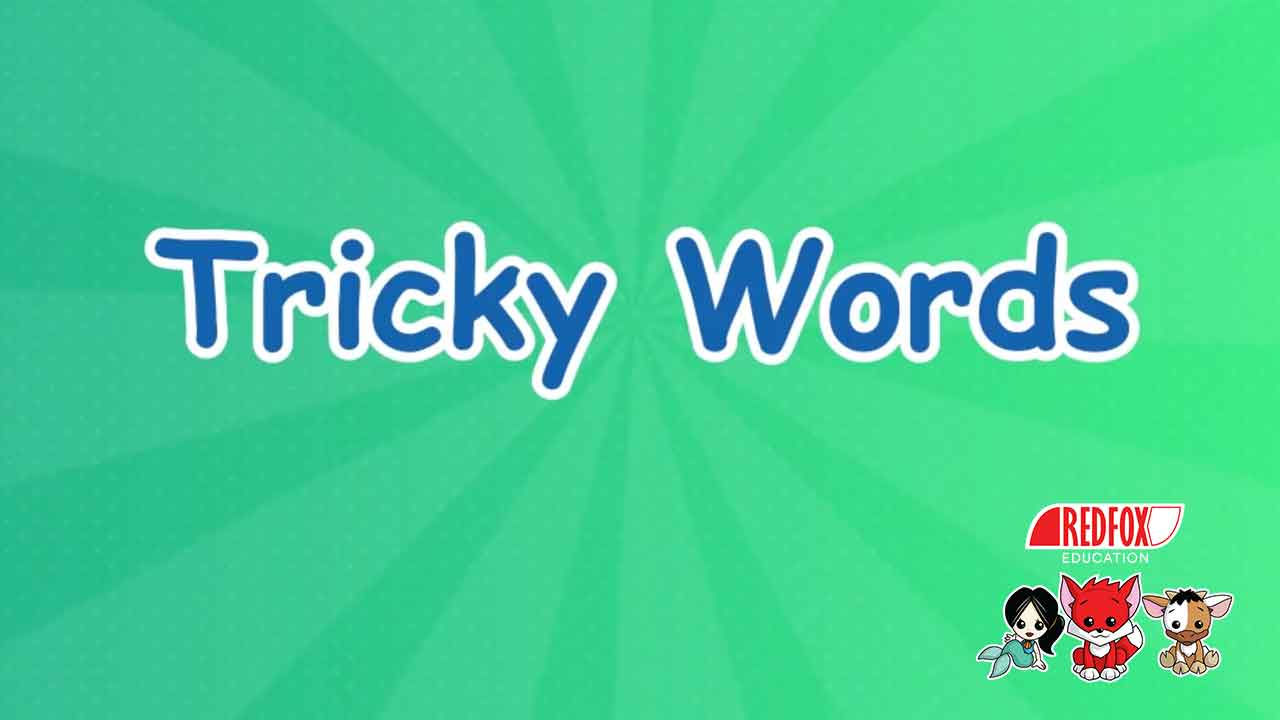##### Let's learn about the Tricky Words
Let's learn about the Tricky Words. What are the tricky words?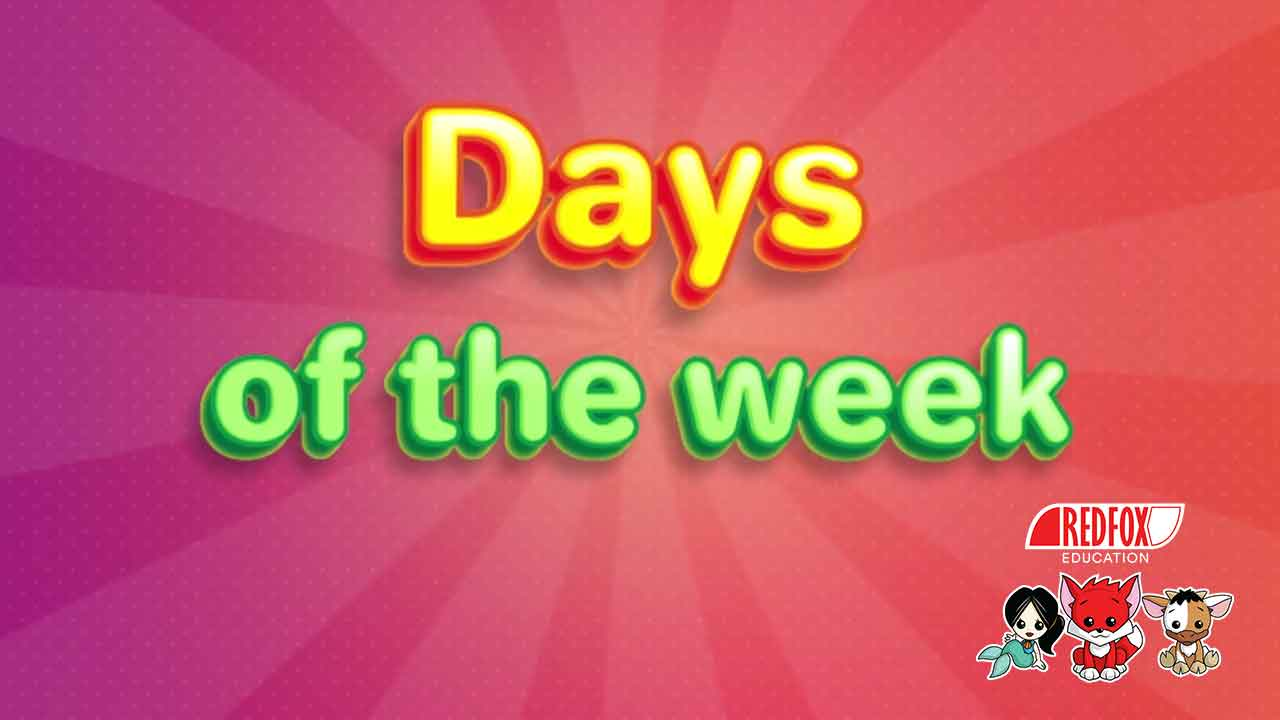##### Let's learn about the Days of the Week
Let's learn about the Days of the Week. What are the days?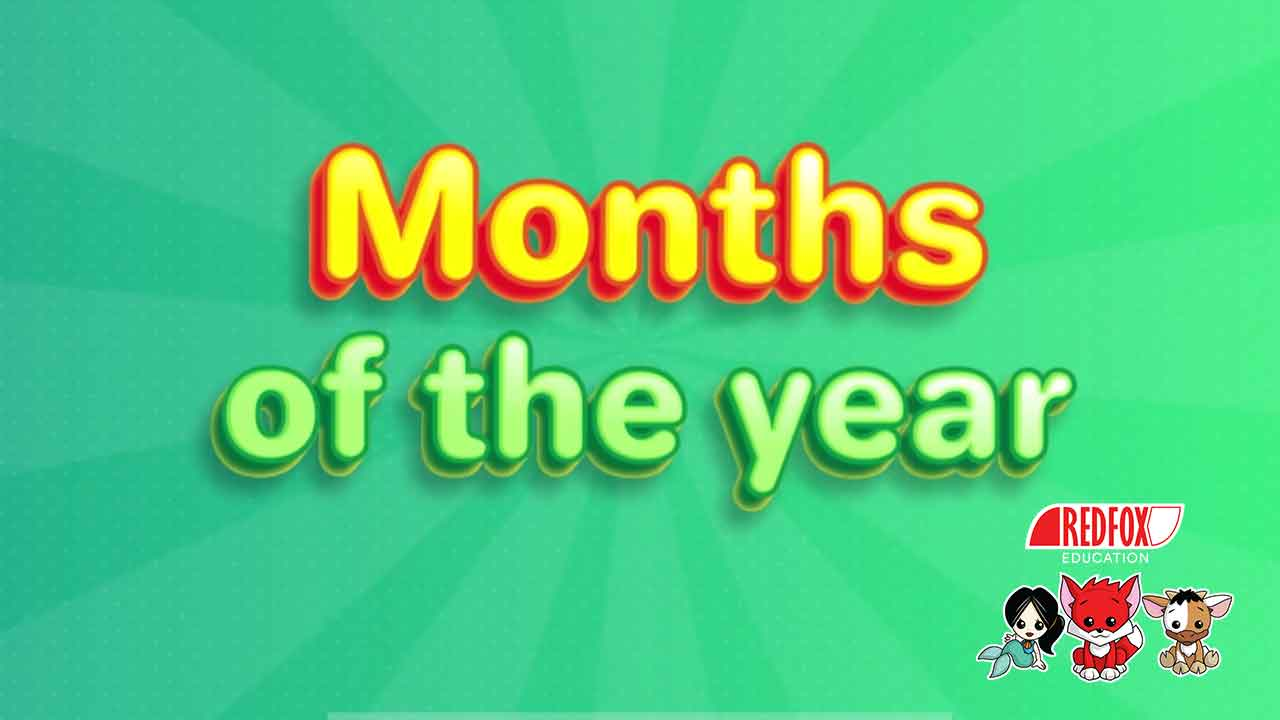##### Let's learn about the Months of the year
Let's learn about the Months of the year. What are months in a year?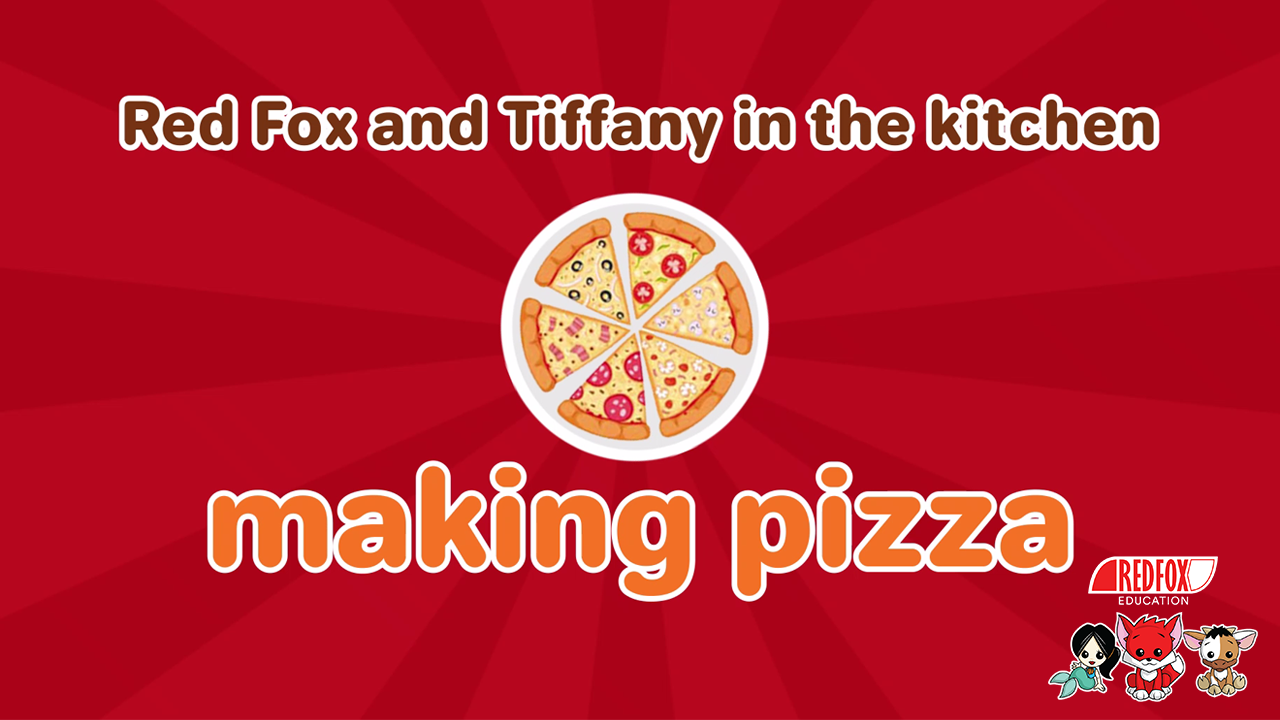##### Let's learn about the Making Pizza
Let's learn about the Making Pizza.How to make?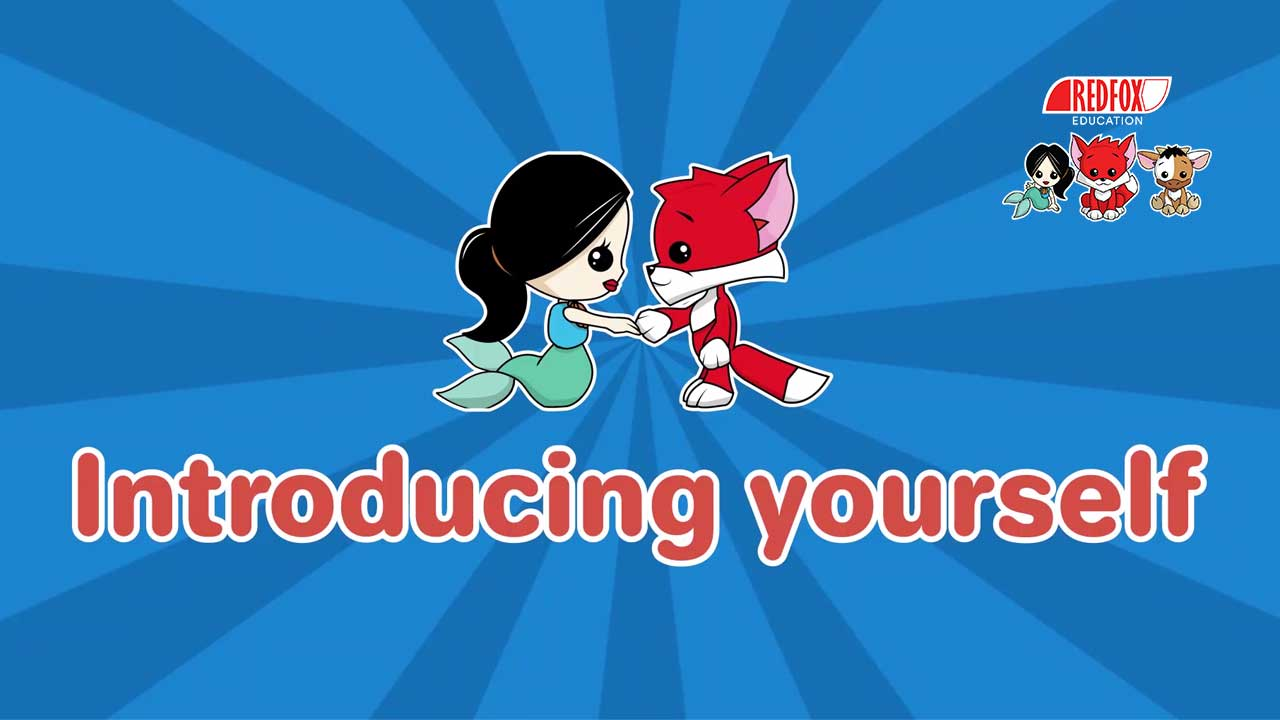##### Let's learn about the Introducing yourself
Let's learn about the Introducing yourself. Who am i?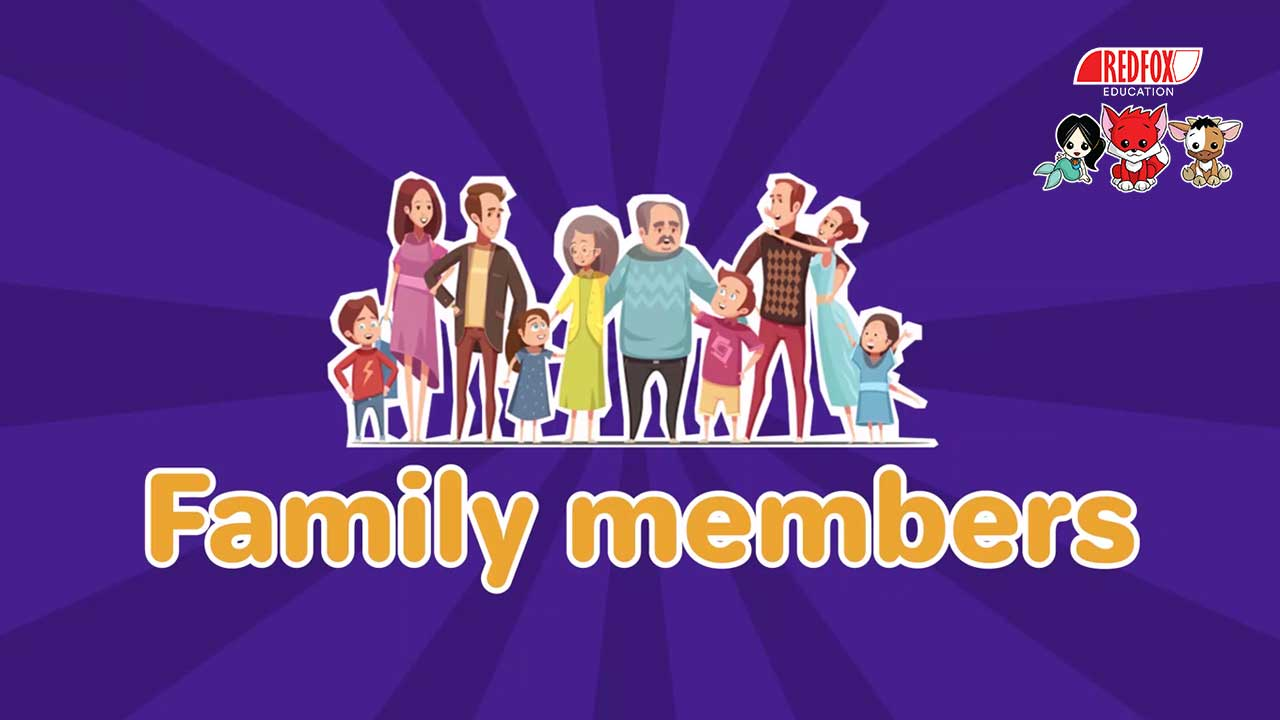##### Let's learn about the Family members
Let's learn about the Family members. Who are all your family members?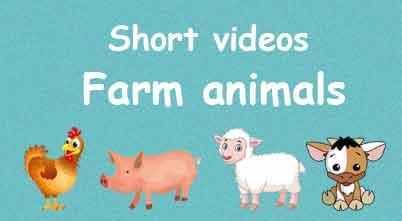##### Let's learn about the Farm Animals
Let's learn about the Farm Animals. Which farm animal do you Know like?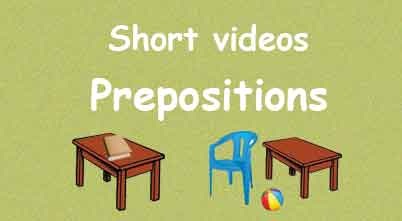##### Let's learn about the Prepositions
Let's learn about the Prepositions. Do you Know prepositions?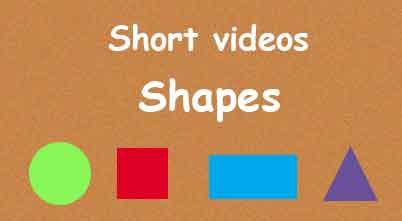##### Let's learn about the Shapes
Let's learn about the Shapes. Which shape do you like?##### Let's learn about the Colours
Let's learn about the Colours. Which colour do you like?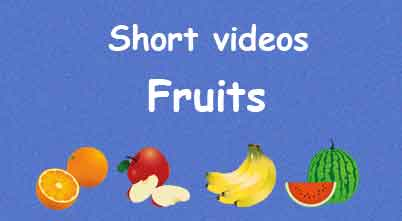##### Let's learn about the Fruits
Let's learn about the Fruits. Which fruit do yo like to eat?